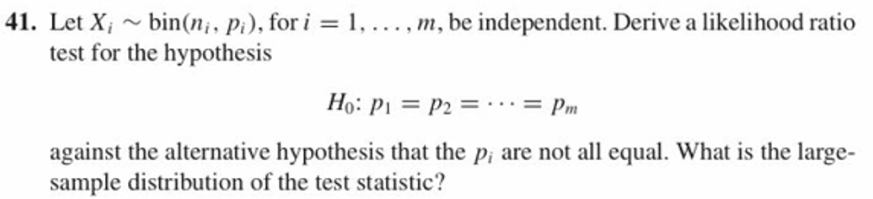41. Let Xi ~ bin(n; , p), for i = I , . . . , m, be independent. Derive a likelihood ratiotest for the hypothesisHo: p1 = p2 = . . . = pmagainst the alternative hypothesis that the p, are not all equal. What is the large-sample distribution of the test statistic?

Question

9.41 question attachedhelp_outlineImage Transcriptionclose41. Let Xi ~ bin(n; , p), for i = I , . . . , m, be independent. Derive a likelihood ratio test for the hypothesis Ho: p1 = p2 = . . . = pm against the alternative hypothesis that the p, are not all equal. What is the large- sample distribution of the test statistic? fullscreen
Step 1

Solution

Given: Let Xi follows bin(

Solution

Given: Let Xi follows bin(ni, pi), for i = 1, 2, …., m, be independent.

We have to derive a likelihood ratio test for the hypothesis H0: p1 = p2 = … = pm against the alternative hypothesis that the pi are not all equal.

ni, pi), for i = 1, 2, …., m, be independent.

We have to derive a likelihood ratio test for the hypothesis H0: p1 = p2 = … = pm against the alternative hypothesis that the pi are not all equal.

Step 2

Step 1:

Since the hypotheses are composite, we have to use the generalized likelihood ratio test

Step 3

The null hypothesis may specify that p ϵ ω0, where ω0 is a subset of the set of all possible values of p, and H1 may specify that p ϵ ω1, ω1 is disjoint from &omega...

Want to see the full answer?

See Solution

Want to see this answer and more?

Our solutions are written by experts, many with advanced degrees, and available 24/7

See Solution
Tagged in

Statistics